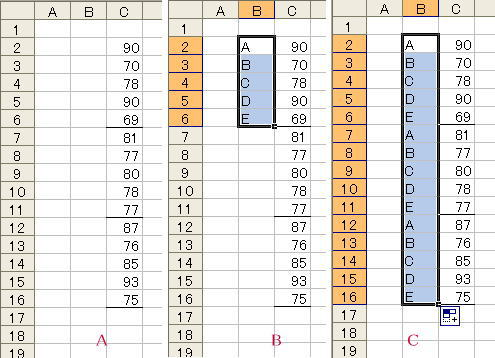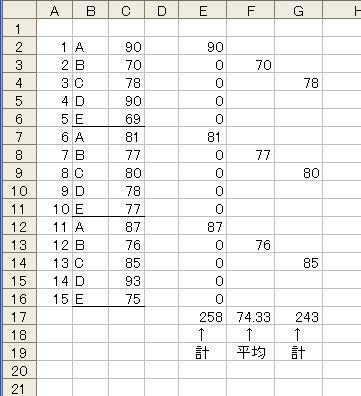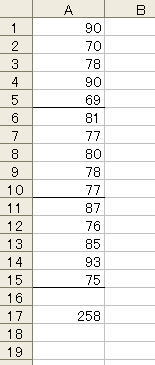Japanese

## Total of Every Fifth line

Here is a proposal to get total amount and average value of items ranging in every fifth line. Total value of the first item in Fig.1 left can be calculated by 『 =c2+c7+c12 』or 『 =sum(c2,c7,c12) 』, and average value of second item is given by 『 =average(c3,c8,c13) 』. But it is very hard if there are so many lines.

### Using Auto-fill technique and SUMIF-function

Fig.1First You type 5 different phrases in B2 to B6 cells. Simple words A, B, C, D and E are chosen here and these are copied entire B column using Auto-fill technique as shown in the Figure 1 right.

You can get total value by typing 『 =sumif(b2：b16, "a", c2：c16) 』 and average value by typing 『 =averageif(b2：b16, "b", c2：c16) 』.

As You cannot use AVERAGEIF-function in Excel2003, utilize another method descrived in the following Section.

Format for SUMIF and AVERAGEIF-function
=SUMIF( Searching range, Searching what, Range for summation )
=AVERAGEIF( Searching range, Searching what, Range for getting average value )
Captal and small letter are not distinguished here for searching.

### Using successive number

Fig.2Prepare a sequenced number in Column A as can be seen in Fig.2.

Content of e2 cell is
『 =if(mod(a2, 5) = 1, c2, 0) 』
This value is copied to all of cells in the range of e3 〜 e16.

Content of e17 cell is
『 =sum(e2：e16) 』
This formula measures the total value of the first item.

『 =mod(a2, 5) 』returns the remainder value when a2 is divided by 5.

On the other hand, Content of f2 cell is
『 =if(mod(a2, 5)= 2, c2, "") 』
and f17 gives the average value of the second item by『 =average(f2：f16) 』.

#### Using Row number

As there is an evident relation between the number of Column A and Row number, it is unnecessary to prepare Column A.
Content of g2 cell in Fig.2 is
『 =if(mod(row() - row(\$c\$2) + 1, 5)= 3, c2, "") 』
The summation value of the third item is determined.

The first Row of Fig.2 is unoccupied. If you prepare a table of no vacancy, the following formula is by far simpler.
『 =if(mod(row(), 5)= 3, c3, "") 』
『 =row() 』 returns Row number of entered cell.

Fig.3If data are filled as like Fig.3,
『 =sumproduct((mod(row(a1：a15), 5) =1)*1, a1：a15) 』
gives a summation of the first item.

[Microsoft Excel help shows] … =SUMPRODUCT(A2：B4, C2：D4) returns a value of [ a2*c2 + b2*d2 + a3*c3 + b3*d3 + a4*c4 + b4*d4 ].

An each element in『 mod(row(a1：a15), 5)=1 』 returns TRUE (value equals 1) if the remainder is 1 when divided by ５, on the other hand, it returns FALSE (value is 0) if the remainder is not 1 (i.e. first item in the table)